0
168

TISSNET Venn Diagram questions with a PDF by Cracku. Practice TISSNET solved Venn Diagram Questions paper tests, and these are the practice question to have a firm grasp on the Venn Diagram topic in the TISSNET exam. Very Important Venn Diagram Questions for TISSNET based on the questions asked in the previous TISSNET exam papers. Click on the link below to download the TISSNET Venn Diagram Questions with answers PDF.

Question 1: Study the given Venn diagram carefully and answer the following question.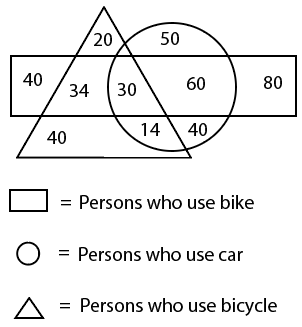How many persons use both a bicycle and a bike but not a car?

a) 30

b) 14

c) 34

d) 40

Solution:

From the Venn diagram,

Number of persons who use both bicycle and bike but not car = Intersection triangle and rectangle but not circle = 34

Hence, the correct answer is Option C

Question 2: Select the Venn diagram that best represents the relationship between the following classes.
Dirham, USD, Currency

a)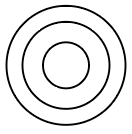b)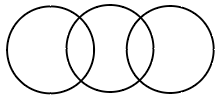c)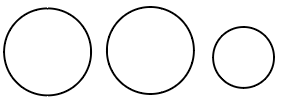d)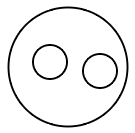Solution:

Dirham and USD are different currencies of different countries.

$\therefore\$The Venn diagram that best represents the relationship between the given classes is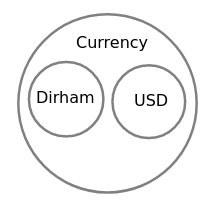Hence, the correct answer is Option D

Question 3: Select the Venn diagram that best represents the relationship between the following classes.
Infants, Males, Fathers

a)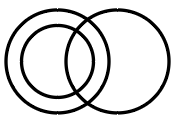b)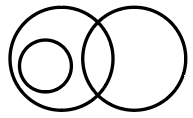c)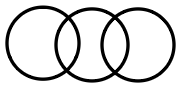d)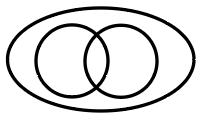Solution:

All Fathers are Males. Some Infants are Males. Infants cannot be Fathers.

$\therefore\$The Venn diagram that best represents the relationship between the given classes is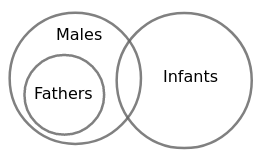Hence, the correct answer is Option B

Question 4: In a batch of 68 students, 23 students do not participate in any of the two games i.e. cricket and squash. 17 students participate in cricket only, 24 students participate in cricket and squash. How many students participate in squash only?

a) 28

b) 4

c) 21

d) 20

Solution:

Let the number of students who participate in squash only = a

The given information is represented in the Venn diagram as shown below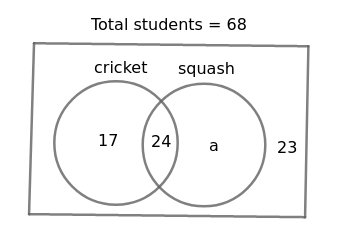From the Venn diagram,

17 + 24 + a + 23 = 68

$\Rightarrow$  64 + a = 68

$\Rightarrow$  a = 4

$\therefore\$Number of students who participate in squash only = 4

Hence, the correct answer is Option B

Question 5: Observe the following diagram and answer the question.
Find the number of students who play any two of the three sports.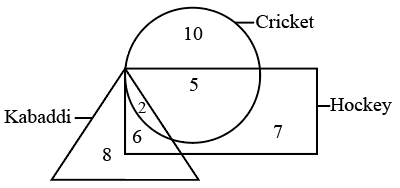a) 9

b) 15

c) 11

d) 13

Solution:

From the diagram,

Number of students who play any two of the three sports = Intersection of circle and rectangle + Intersection of triangle and rectangle = 5 + 6 = 11

Hence, the correct answer is Option C

Question 6: Select the Venn diagram that best illustrates the relationship between the following classes.
Fathers, Males, Brothers

a)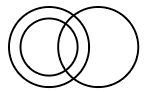b)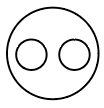c)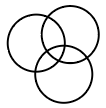d)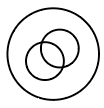Solution:

All fathers and brothers are males. Some fathers are brothers.

$\therefore\$The Venn diagram that best illustrates the relationship between the given classes is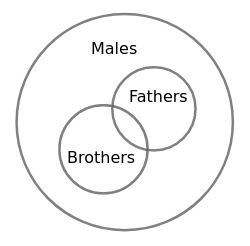Hence, the correct answer is Option D

Question 7: In the given Venn Diagram, ‘Circle’ represents ‘Players’, ‘Triangle’ represents ‘Graduates’ and ‘Rectangle’ represents ‘Managers’. Which of the numbers depicts the area which represents ‘Graduate Players who are not Managers’?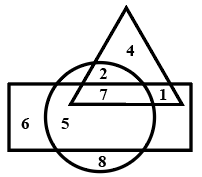a) 1

b) 5

c) 7

d) 2

Solution:

From the Venn diagram,

The number which represents ‘Graduate Players who are not Managers’ = Intersection of Triangle and Circle but not Rectangle = 2

Hence, the correct answer is Option D

Question 8: Select the Venn diagram that best represents the relationship between the following classes.
Physics, Science, Biology, Zoology

a)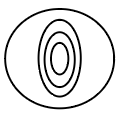b)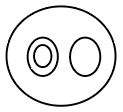c)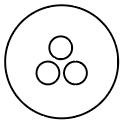d)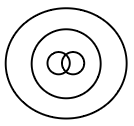Solution:

Physics, Biology comes under Science and Zoology comes under Biology.

$\therefore\$The Venn diagram that best represents the relationship between the given classes is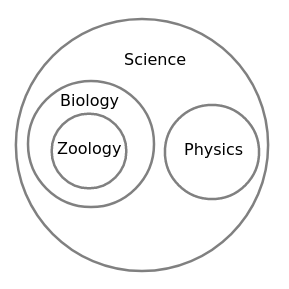Hence, the correct answer is Option B

Question 9: Select the Venn diagram that best represents the relationship between the following classes.
Tortoise, Mammal, Amphibian

a)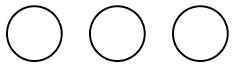b)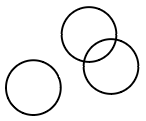c)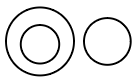d)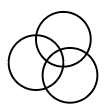Solution:

Mammals are the ones that feed their younger ones with milk.

Amphibians are the ones that can live both in land and water.

Tortoise is neither Mammal nor Amphibian.

$\therefore\$The Venn diagram that best represents the relationship between the given classes is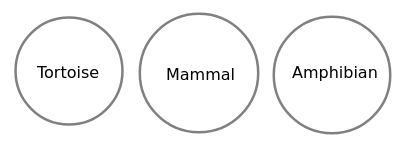Hence, the correct answer is Option A

Question 10: Study the following diagram and answer the given question.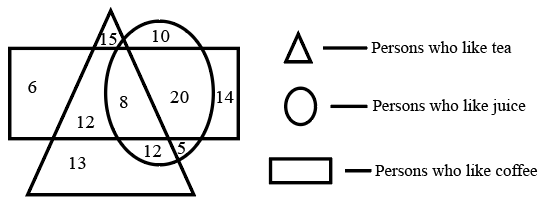How many people like both tea and coffee, but do NOT like juice?’

a) 22

b) 12

c) 8

d) 20

Solution:

From the diagram,

Number of people like both tea and coffee, but do not like juice = Intersection of triangle and rectangle but not circle = 12

Hence, the correct answer is Option B

Question 11: In the given Venn diagram, A likes milk, B likes bread and C likes meat. Who likes all the given eating materials?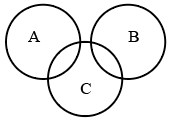a) Only B

b) Only C

c) Both B and C

d) Both A and B

Solution:

From the Venn diagram,

A has intersection with only C, so A likes milk and meat.

B has intersection with only C, so B likes bread and meat.

C has intersection with both A and B, so C likes meat, milk, bread.

$\therefore\$Only C likes all the given eating materials

Hence, the correct answer is Option B

Question 12: In the given Venn diagram, circle A represents books, circle B represents pens and circle C, represents pencils. Which number represents only books and pencils?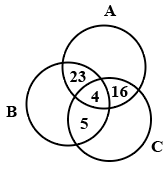a) 16

b) 23

c) 5

d) 20

Solution:

From the Venn Diagram,

Number which represents only books and pencils = Intersection of A and C but not B = 16

Hence, the correct answer is Option A

Question 13: In the given Venn diagram, A denotes mangoes, B denotes bananas, C denotes apples, and the numbers in each section represent the number of persons who like those fruits. How many persons like only bananas?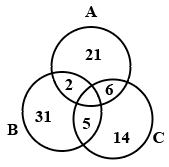a) 38

b) 7

c) 31

d) 33

Solution:

From the Venn diagram,

Persons who like only bananas = Only B without intersection of A and C = 31

Hence, the correct answer is Option C

Question 14: Study the following diagram.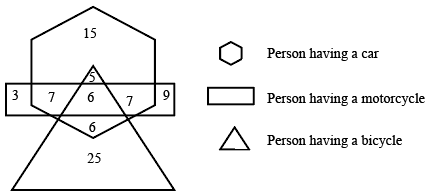How many persons have only motorcycle?

a) 32

b) 3

c) 9

d) 12

Solution:

From the given diagram,

Number of persons who have only motorcycle = Number of persons in rectangle with no intersection with hexagon and triangle = 3 + 9 = 12

Hence, the correct answer is Option D

Question 15: In the given Venn diagram, different animals are represented by different areas. ‘A’ denotes ‘dogs’, ‘B’ denotes ‘cats’, ‘C’ denotes ‘fishes’, ‘D’ denotes ‘mongooses’, ‘E’ denotes ‘rats’, and ‘F’ denotes ‘rabbits’. Which area represents the group of only dogs, fishes and mongooses?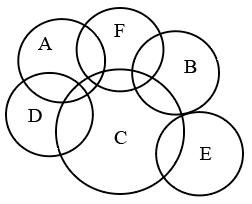a) D

b) F

c) C

d) A

Solution:

From the Venn diagram,

D has an intersection with only A and C, so D contains only dogs, fishes and mongooses. All other letters contain other animals.

Hence, the correct answer is Option A

Question 16: In the given Venn diagram, different areas denote different types of players. ‘A’ denotes ‘tennis players’, ‘B’ denotes ‘badminton players’, ‘C’ denotes ‘hockey players’ and ‘D’ denotes‘football players’. Which area represents the group of all types of sports players?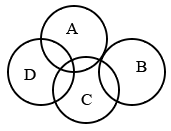a) B

b) D

c) A

d) C

Solution:

From the Venn diagram,

C has an intersection with A, B, D

$\Rightarrow$ C consists of players who can play Tennis, Badminton, Hockey, Football

$\therefore\$C represents the group of all types of sports players

Hence, the correct answer is Option D

Question 17: Select the Venn diagram that best represents the relationship between the following words.

a)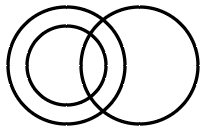b)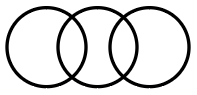c)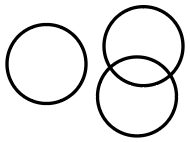d)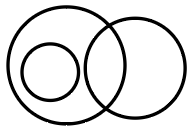Solution:

$\therefore\$The Venn diagram that best represents the relationship between the given words is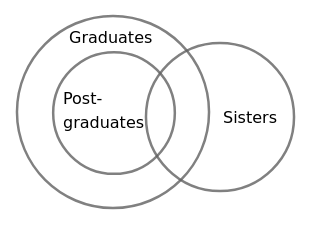Hence, the correct answer is Option A

Question 18: Consider the following diagram.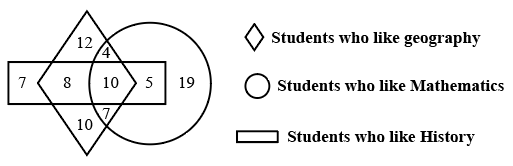How many students like both geography and mathematics but NOT history?

a) 21

b) 4

c) 7

d) 11

Solution:

From the diagram,

Number of students like both geography and mathematics but not history = Intersection of Rhombus and Circle but not Rectangle = 4 + 7 = 11

Hence, the correct answer is Option D

Question 19: Select the Venn diagram that best represents the relationship between the following classes.
Animals, Cows, Dogs

a)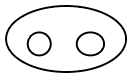b)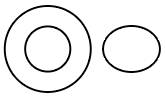c)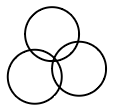d)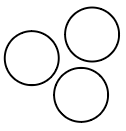Solution:

Cows and Dogs are different Animals.

$\therefore\$The Venn diagram that best represents the relationship between the following classes is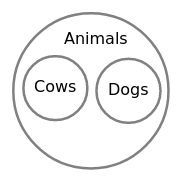Hence, the correct answer is Option A

Question 20: In the given Venn Diagram, if the ‘circle’ represents ‘senior lawyers’, the ‘triangle’ represents ‘fathers’ and the ‘rectangle’ represents ‘Indians’, then how many Indian senior lawyers are there who are NOT fathers?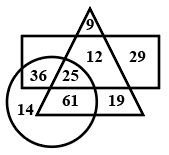a) 12

b) 61

c) 25

d) 36